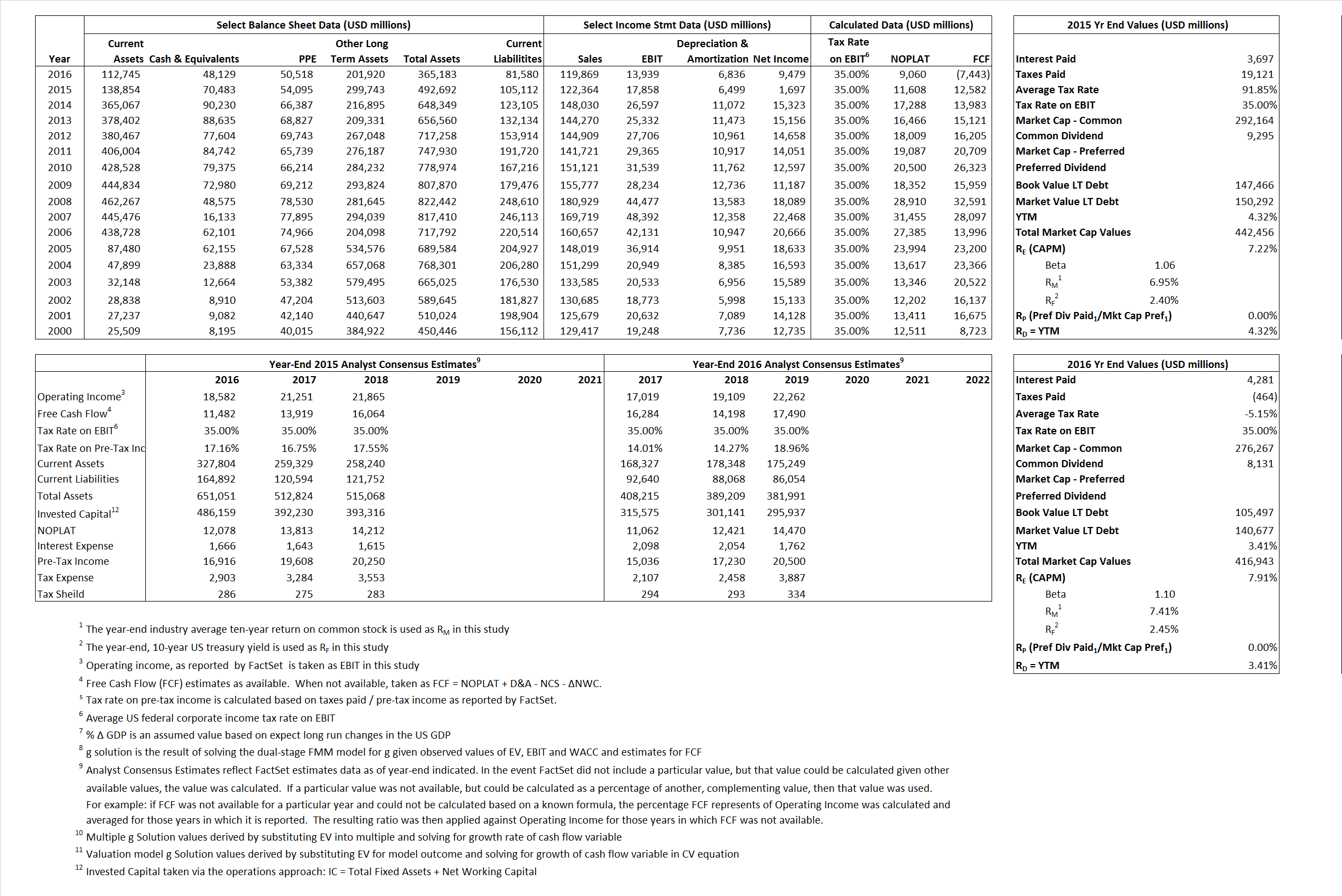# General Eletric

## Analyst Listing

The following analysts provide coverage for the subject firm as of May 2016:

 Broker Analyst Analyst Email Stifel Nicolaus Robert P. McCarthy mccarthyr@stifel.com Credit Suisse Julian Mitchell julian.mitchell@credit-suisse.com Bernstein Research Steven E. Winoker steven.winoker@bernstein.com Deutsche Bank Research John G. Inch john.inch@db.com RBC Capital Markets Deane M. Dray deane.dray@rbccm.com Oppenheimer Christopher Glynn christopher.glynn@opco.com William Blair Nicholas P. Heymann nheymann@williamblair.com Langenberg, LLC Brian K. Langenberg brian@langenberg-llc.com

## Primary Input Data## Derived Input Data

### Equational Form

Net Operating Profit Less Adjusted Taxes NOPLAT  11,608 9,060$NOPLAT\, =\, EBIT\, x\, (1 \,-\, Avg \,\,Tax\,\, Rate\,\, on\,\, EBIT)$
Free Cash Flow FCF  12,582  (7,443)$FCF\,=NOPLAT\,+\,Non-Cash\,Expenses-\Delta NWC\,-\,NCS$
Tax Shield TS  3,396 (220)$TS\,=\,Interest\,\,Paid\,\,x\,\, Avg \,\,Tax\,\,Rate\,\, on\,\, Pre-Tax\,\, Income$
Invested Capital IC 387,580 283,603$IC\,=\,Fixed\,\,Operating\,\,Assets\,\,+\,\,Net\,\, Working\,\, Capital$
Return on Invested Capital ROIC 2.99% 3.19%$ROIC\,=\,\frac { NOPLAT }{ IC }$
Net Investment NetInv (131,165) (97,141)$NetInv\,=\,{ {IC}_{1}}-{{IC}_{0}}+Depreciation$
Investment Rate IR -1,129.98% -1,072.16%$IR\,=\,\frac {NetInv}{NOPLAT}$
Weighted Average Cost of Capital
WACCMarket  4.89% 6.45%$WACC\,=\,\frac { E }{ V } { R }_{ E }\,+\,\frac { P }{ V } { R }_{ P }\,+\,\frac { D }{ V } { R }_{ D }\left( 1- Avg\,\, Tax\,\,Rate\,\,on\,\,Pre-Tax\,\,Income \right)$
WACCBook     6.23%     7.43%
Enterprise value
EVMarket  371,973  368,814$EV\,=\,Market\,\,Cap\,\,Equity\,+\,\,Long\,\,Term\,\,Debt\,-\,Cash$
EVBook    369,147    333,635
Long-Run Growth
g = IR x ROIC
-33.84%    -34.25% Long-run growth rates of the income variable are used in the Continuing Value portion of the valuation models.
g = %$\Delta$ GDP     2.50%     2.50%
Margin from Operations M    14.59%    11.63%$M\,\,=\,\,\frac{EBIT}{SALES}$
Depreciation/Amortization Rate D    26.68%    32.90%$D\,\,=\,\,\frac{D+A}{EBITDA}$

## Valuation Multiple Outcomes

The outcomes presented in this study are the result of original input data, derived data, and synthesized inputs.

### model g solution

12/31/2015 12/31/2016 12/31/2015 12/31/2016 12/31/2015 12/31/2016

EV/SALES$\frac {EV}{Sales} \,= \,\frac{ROIC\, -\, g}{ROIC\,(WACC\,-\,g)}\,(1\,-\,T)\,(M)$

3.04  3.08  -42.15%  17.28%  -444.48%  12.17%

EV/EBITDA$\frac {EV}{EBITDA} \,= \,\frac{ROIC\, -\, g}{ROIC\,(WACC\,-\,g)}\,(1\,-\,T)\,(1\,-\,D)$

15.27 17.75 -42.15% 17.28% -444.48% 12.17%

EV/NOPLAT$\frac {EV}{NOPLAT} \,= \,\frac{ROIC\, -\, g}{ROIC\,(WACC\,-\,g)}$

32.05 40.71 -42.15% 17.28% -444.48% 12.17%

EV/FCFOPS$\frac {EV}{FCF_{OPS}} \,= \,\frac{ROIC\, -\, g}{ROIC\,(WACC\,-\,g)}\,(1\,-\,T)$

29.56 -49.55 -42.15% 17.28% -444.48%  12.17%

EV/EBIT$\frac {EV}{EBIT} \,= \,\frac{ROIC\, -\, g}{ROIC\,(WACC\,-\,g)}\,(1\,-\,T)$

20.83 26.46 -42.15% 17.28% -444.48% 12.17%

EV/IC$\frac {EV}{IC} \,= \,\frac{ROIC\, -\, g}{WACC\,-\,g}$

0.96  1.30 C-42.15% 17.28% -444.48% 12.17%